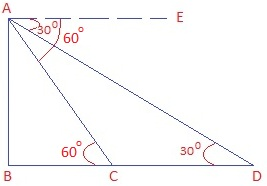Guru

# A straight highway leads to the foot of a tower. A man standing at the top of the tower observes a car at an angle of depression of 30°, which is approaching the foot of the tower with a uniform speed. Six seconds later, the angle of depression of the car is found to be 60°. Find the time taken by the car to reach the foot of the tower from this point. Q.15

• 0

What is the way for solving this tough question from class 10th math of chapter Some Applications of Trigonometry of Exercise 9.1 of question 15, how i solve this problem because it is very important for board exams A straight highway leads to the foot of a tower. A man standing at the top of the tower observes a car at an angle of depression of 30°, which is approaching the foot of the tower with a uniform speed. Six seconds later, the angle of depression of the car is found to be 60°. Find the time taken by the car to reach the foot of the tower from this point.

Share

1. Let AB be the tower.

D is the initial and C is the final position of the car respectively.

Since man is standing at the top of the tower so, Angles of depression are measured from A.

BC is the distance from the foot of the tower to the car.Step 1: In right ΔABC,

tan 60° = AB/BC

√3 = AB/BC

BC = AB/√3

AB = √3 BC

Step 2:

In right ΔABD,

tan 30° = AB/BD

1/√3 = AB/BD

AB = BD/√3

Step 3: Form step 1 and Step 2, we have

√3 BC = BD/√3 (Since LHS are same, so RHS are also same)

3 BC = BD

3 BC = BC + CD

2BC = CD

or BC = CD/2

Here, distance of BC is half of CD. Thus, the time taken is also half.

Time taken by car to travel distance CD = 6 sec. Time taken by car to travel BC = 6/2 = 3 sec.

• 0